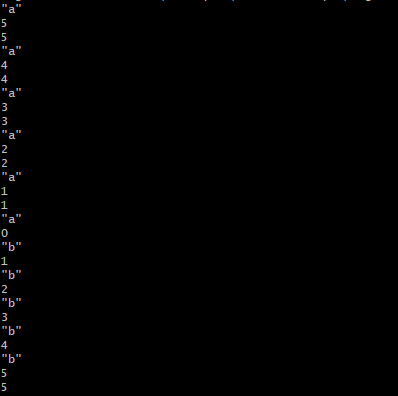# [RUBY] Aiming for a basic understanding of the flow of recursive processing

Even if I read the code description about the recursive function that "calls myself", I couldn't catch up with it, so I wrote an article with the theme of "aiming for a basic understanding of the flow of recursive processing". I would like to try. Let's think about how the function is executed using the simple code below.

#### Recursive function

``````1  def recursive(n)
2     p 'a'
3     p n
4     return 1 if n == 0
5     p n
6     n * recursive(n - 1)
7     p 'b'
8     p n
9  end
10
11  p recursive(5)
``````

When executed, it is as follows.At first glance, I was confused because I had no idea why the numbers increased from 0. While imagining that "a function contains a function", I will try to output the mechanism of the recursive function by chewing it in my own way as follows.

#### Rough flow

(1) Function execution given 5 as an argument → (2) p "a" to p n on the 5th line are executed (* Of course, the postfix if statement return is not executed) → (3) On the 6th line, its own function recursive (4) is executed. → Like the (4) loop, the above (2) to (3) are executed up to recursive (0). (* At this point, "(omitted) ..." a ", 1, 1" has been processed. ") → (5) When recursive (0) is executed, the postfix if statement return is executed after p "a" to p n on the third line are executed. With return, recursive (0) function processing ends. → (6) Rewind from here? Retrograde? It becomes a state like. The execution scene returns to recursive (1), "b" on the 7th line and p n (* n is 1) on the 8th line are executed, and recursive (1) ends. → (7) The execution scene returns to recursive (2), "b" on the 7th line and p n (* n is 2) on the 8th line are executed, and recursive (2) ends. → (Omitted up to recursive (4)) → (8) The execution scene returns to recursive (5), "b" on the 7th line and p n (* n is 5) on the 8th line are executed, recursive (5) ends, and all functions are completed. → (9) Finally, p recursive (5) (synonymous with p n) 5 is output and the process ends. (* If the p method is excluded, the last 5 will not be output.)

... Personally, recursive functions are quite difficult to understand. I'm a little worried about whether it's better to manage the number of places and get used to it, and write a brush. : confused: